Скачать презентацию Planning Adapted from Tim Finin UMBC Marie des

0f09913180bcdea1d2456e310cafa848.ppt

• Количество слайдов: 27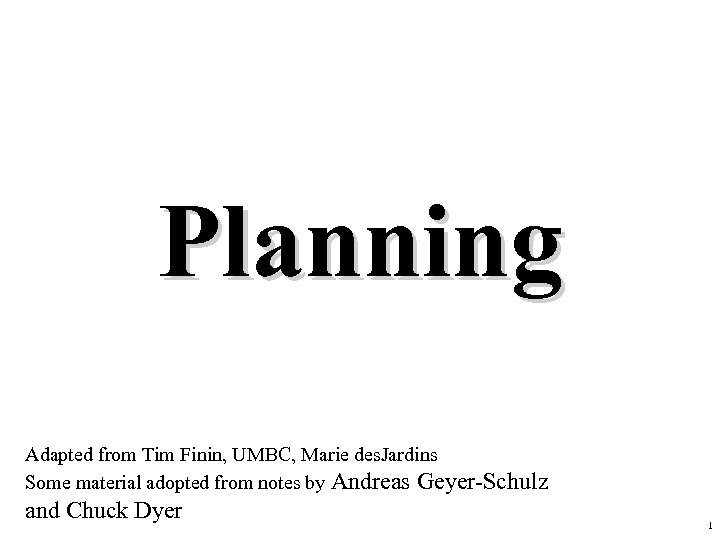Planning Adapted from Tim Finin, UMBC, Marie des. Jardins Some material adopted from notes by Andreas Geyer-Schulz and Chuck Dyer 1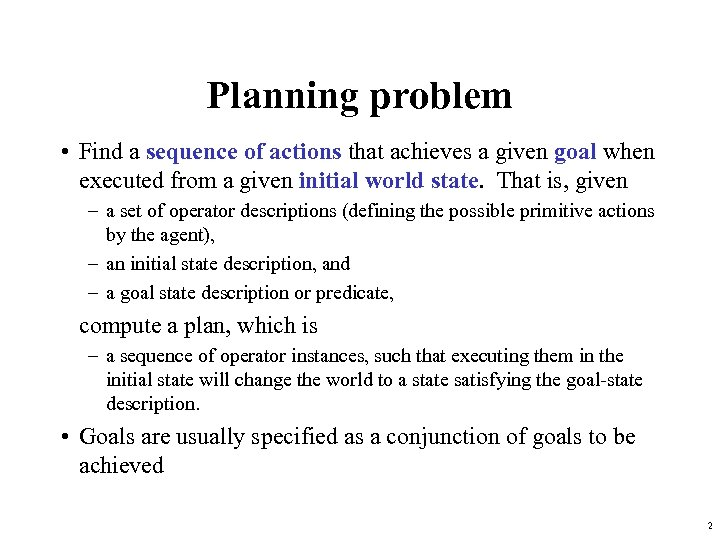Planning problem • Find a sequence of actions that achieves a given goal when executed from a given initial world state. That is, given – a set of operator descriptions (defining the possible primitive actions by the agent), – an initial state description, and – a goal state description or predicate, compute a plan, which is – a sequence of operator instances, such that executing them in the initial state will change the world to a state satisfying the goal-state description. • Goals are usually specified as a conjunction of goals to be achieved 2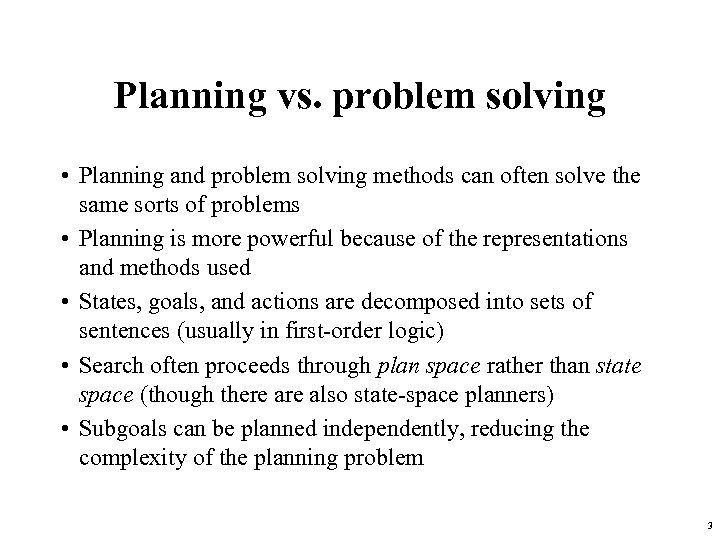Planning vs. problem solving • Planning and problem solving methods can often solve the same sorts of problems • Planning is more powerful because of the representations and methods used • States, goals, and actions are decomposed into sets of sentences (usually in first-order logic) • Search often proceeds through plan space rather than state space (though there also state-space planners) • Subgoals can be planned independently, reducing the complexity of the planning problem 3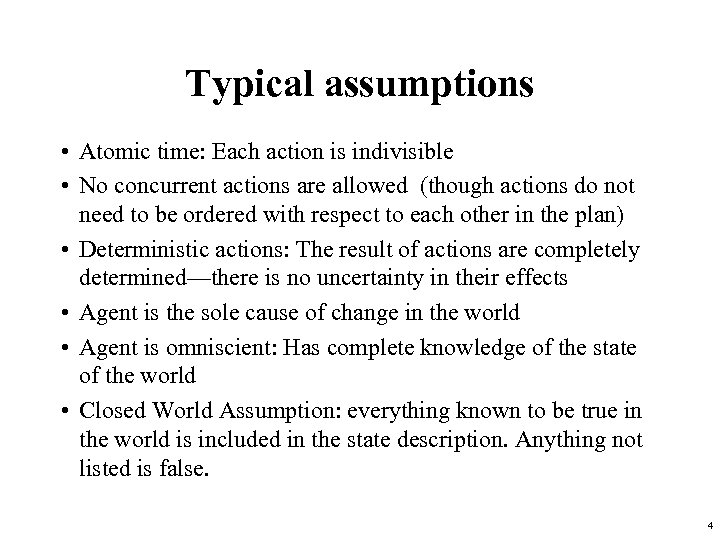Typical assumptions • Atomic time: Each action is indivisible • No concurrent actions are allowed (though actions do not need to be ordered with respect to each other in the plan) • Deterministic actions: The result of actions are completely determined—there is no uncertainty in their effects • Agent is the sole cause of change in the world • Agent is omniscient: Has complete knowledge of the state of the world • Closed World Assumption: everything known to be true in the world is included in the state description. Anything not listed is false. 4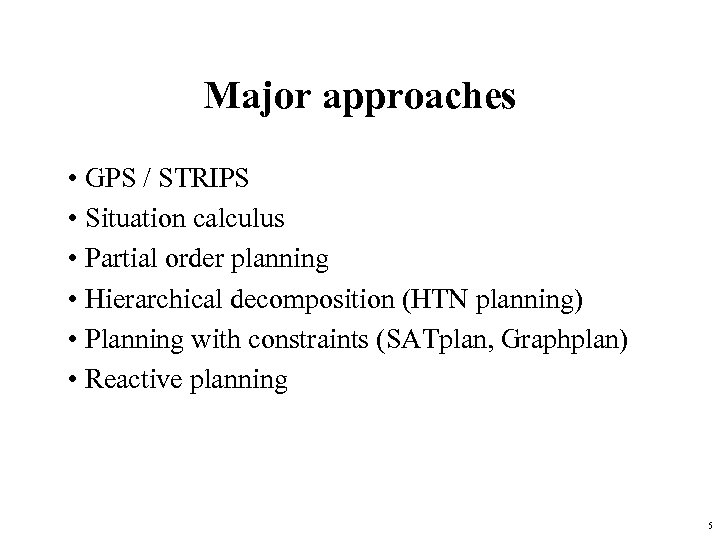Major approaches • GPS / STRIPS • Situation calculus • Partial order planning • Hierarchical decomposition (HTN planning) • Planning with constraints (SATplan, Graphplan) • Reactive planning 5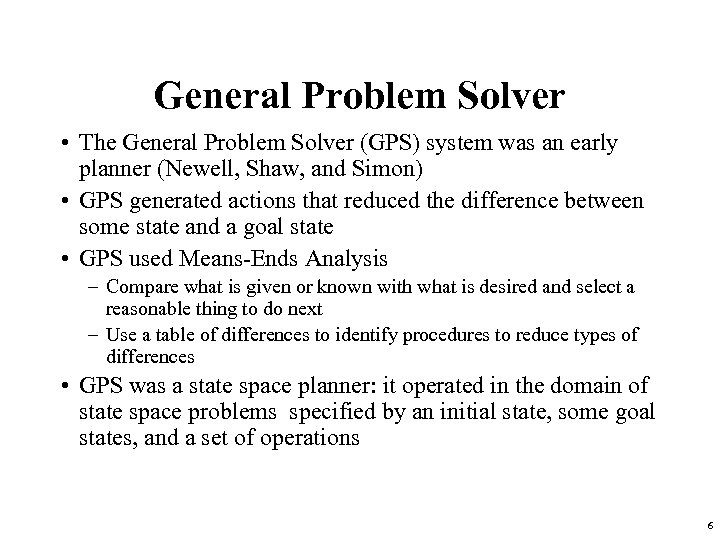General Problem Solver • The General Problem Solver (GPS) system was an early planner (Newell, Shaw, and Simon) • GPS generated actions that reduced the difference between some state and a goal state • GPS used Means-Ends Analysis – Compare what is given or known with what is desired and select a reasonable thing to do next – Use a table of differences to identify procedures to reduce types of differences • GPS was a state space planner: it operated in the domain of state space problems specified by an initial state, some goal states, and a set of operations 6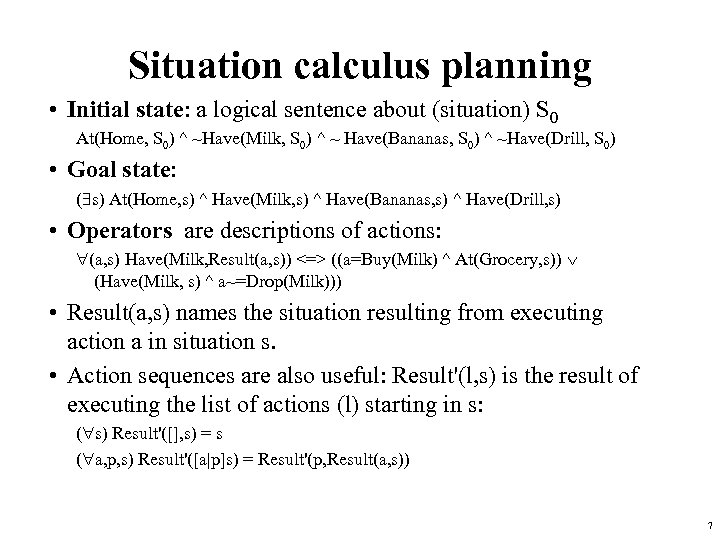Situation calculus planning • Initial state: a logical sentence about (situation) S 0 At(Home, S 0) ^ ~Have(Milk, S 0) ^ ~ Have(Bananas, S 0) ^ ~Have(Drill, S 0) • Goal state: ( s) At(Home, s) ^ Have(Milk, s) ^ Have(Bananas, s) ^ Have(Drill, s) • Operators are descriptions of actions: (a, s) Have(Milk, Result(a, s)) <=> ((a=Buy(Milk) ^ At(Grocery, s)) (Have(Milk, s) ^ a~=Drop(Milk))) • Result(a, s) names the situation resulting from executing action a in situation s. • Action sequences are also useful: Result'(l, s) is the result of executing the list of actions (l) starting in s: ( s) Result'([], s) = s ( a, p, s) Result'([a|p]s) = Result'(p, Result(a, s)) 7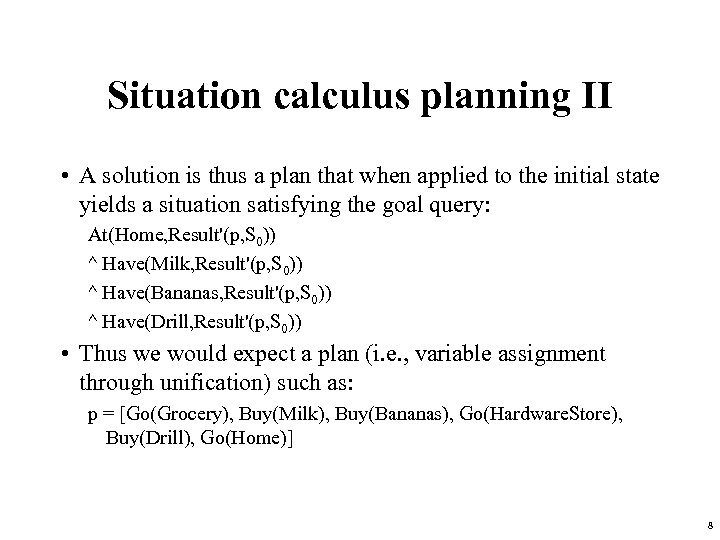Situation calculus planning II • A solution is thus a plan that when applied to the initial state yields a situation satisfying the goal query: At(Home, Result'(p, S 0)) ^ Have(Milk, Result'(p, S 0)) ^ Have(Bananas, Result'(p, S 0)) ^ Have(Drill, Result'(p, S 0)) • Thus we would expect a plan (i. e. , variable assignment through unification) such as: p = [Go(Grocery), Buy(Milk), Buy(Bananas), Go(Hardware. Store), Buy(Drill), Go(Home)] 8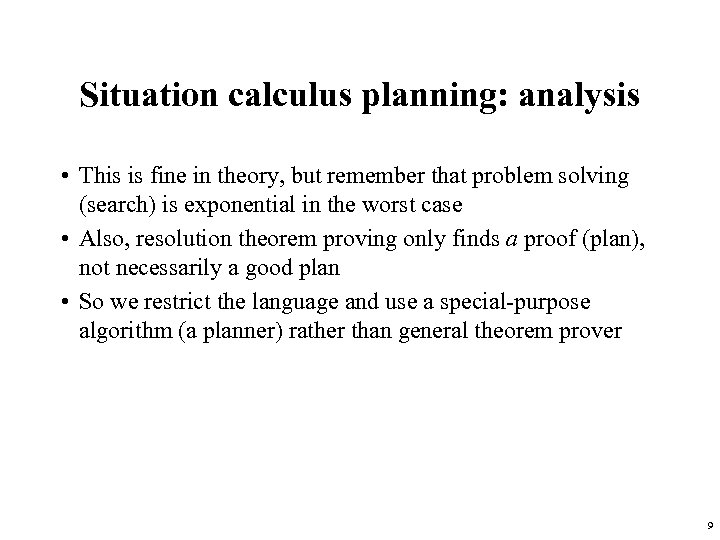Situation calculus planning: analysis • This is fine in theory, but remember that problem solving (search) is exponential in the worst case • Also, resolution theorem proving only finds a proof (plan), not necessarily a good plan • So we restrict the language and use a special-purpose algorithm (a planner) rather than general theorem prover 9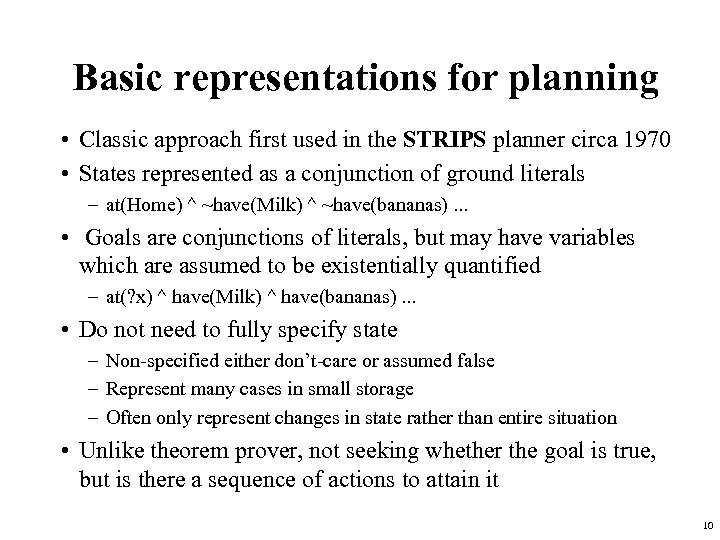Basic representations for planning • Classic approach first used in the STRIPS planner circa 1970 • States represented as a conjunction of ground literals – at(Home) ^ ~have(Milk) ^ ~have(bananas). . . • Goals are conjunctions of literals, but may have variables which are assumed to be existentially quantified – at(? x) ^ have(Milk) ^ have(bananas). . . • Do not need to fully specify state – Non-specified either don’t-care or assumed false – Represent many cases in small storage – Often only represent changes in state rather than entire situation • Unlike theorem prover, not seeking whether the goal is true, but is there a sequence of actions to attain it 10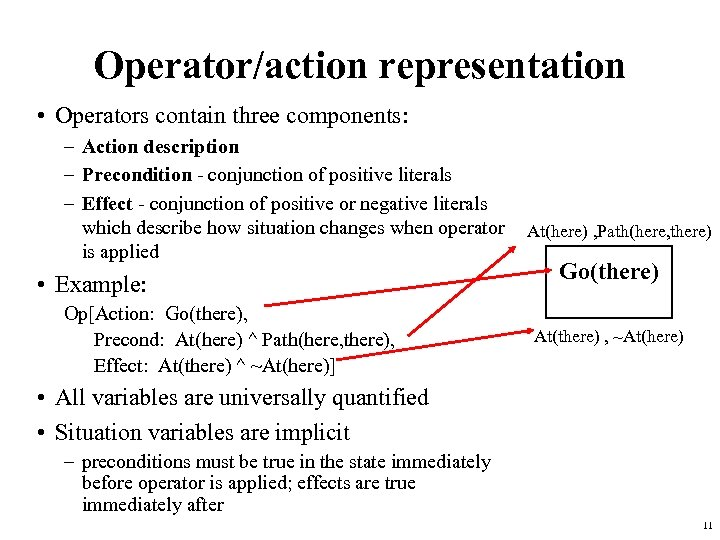Operator/action representation • Operators contain three components: – Action description – Precondition - conjunction of positive literals – Effect - conjunction of positive or negative literals which describe how situation changes when operator is applied • Example: Op[Action: Go(there), Precond: At(here) ^ Path(here, there), Effect: At(there) ^ ~At(here)] At(here) , Path(here, there) Go(there) At(there) , ~At(here) • All variables are universally quantified • Situation variables are implicit – preconditions must be true in the state immediately before operator is applied; effects are true immediately after 11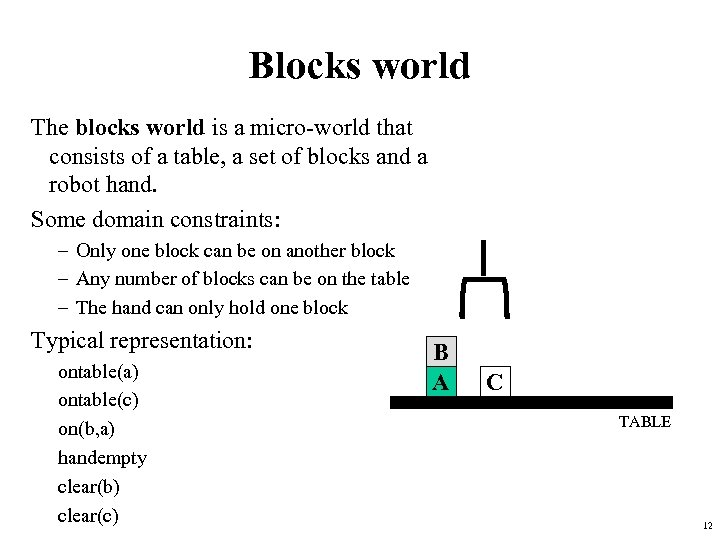Blocks world The blocks world is a micro-world that consists of a table, a set of blocks and a robot hand. Some domain constraints: – Only one block can be on another block – Any number of blocks can be on the table – The hand can only hold one block Typical representation: ontable(a) ontable(c) on(b, a) handempty clear(b) clear(c) B A C TABLE 12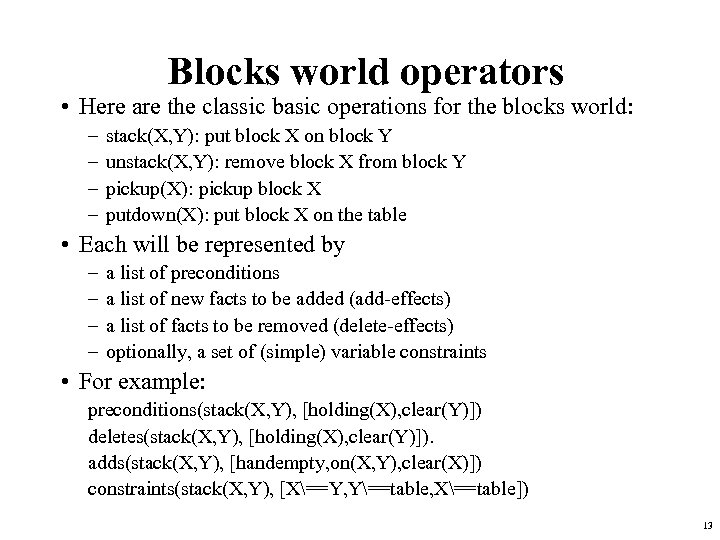Blocks world operators • Here are the classic basic operations for the blocks world: – – stack(X, Y): put block X on block Y unstack(X, Y): remove block X from block Y pickup(X): pickup block X putdown(X): put block X on the table • Each will be represented by – – a list of preconditions a list of new facts to be added (add-effects) a list of facts to be removed (delete-effects) optionally, a set of (simple) variable constraints • For example: preconditions(stack(X, Y), [holding(X), clear(Y)]) deletes(stack(X, Y), [holding(X), clear(Y)]). adds(stack(X, Y), [handempty, on(X, Y), clear(X)]) constraints(stack(X, Y), [X==Y, Y==table, X==table]) 13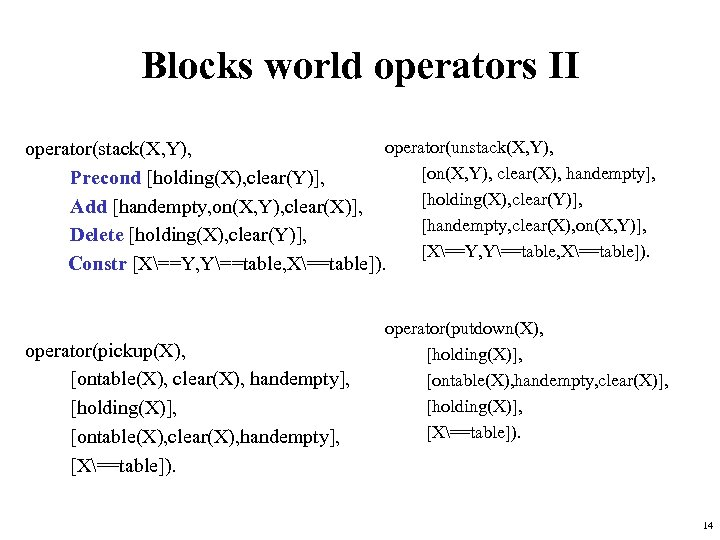Blocks world operators II operator(unstack(X, Y), operator(stack(X, Y), [on(X, Y), clear(X), handempty], Precond [holding(X), clear(Y)], Add [handempty, on(X, Y), clear(X)], [handempty, clear(X), on(X, Y)], Delete [holding(X), clear(Y)], [X==Y, Y==table, X==table]). Constr [X==Y, Y==table, X==table]). operator(pickup(X), [ontable(X), clear(X), handempty], [holding(X)], [ontable(X), clear(X), handempty], [X==table]). operator(putdown(X), [holding(X)], [ontable(X), handempty, clear(X)], [holding(X)], [X==table]). 14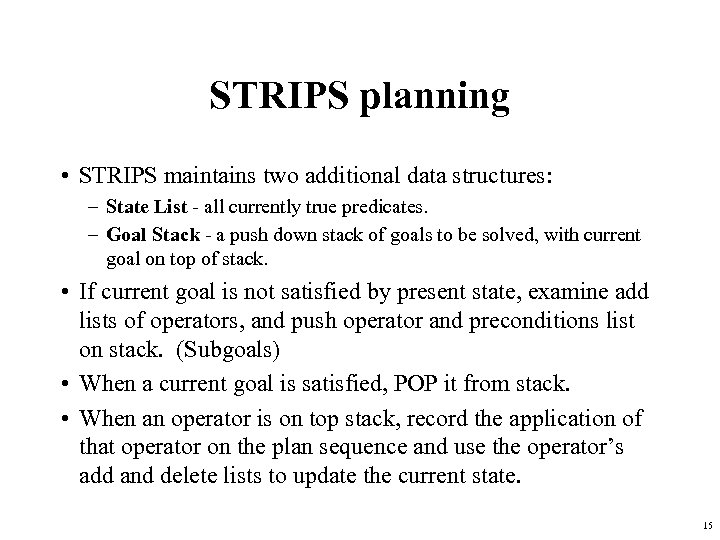STRIPS planning • STRIPS maintains two additional data structures: – State List - all currently true predicates. – Goal Stack - a push down stack of goals to be solved, with current goal on top of stack. • If current goal is not satisfied by present state, examine add lists of operators, and push operator and preconditions list on stack. (Subgoals) • When a current goal is satisfied, POP it from stack. • When an operator is on top stack, record the application of that operator on the plan sequence and use the operator’s add and delete lists to update the current state. 15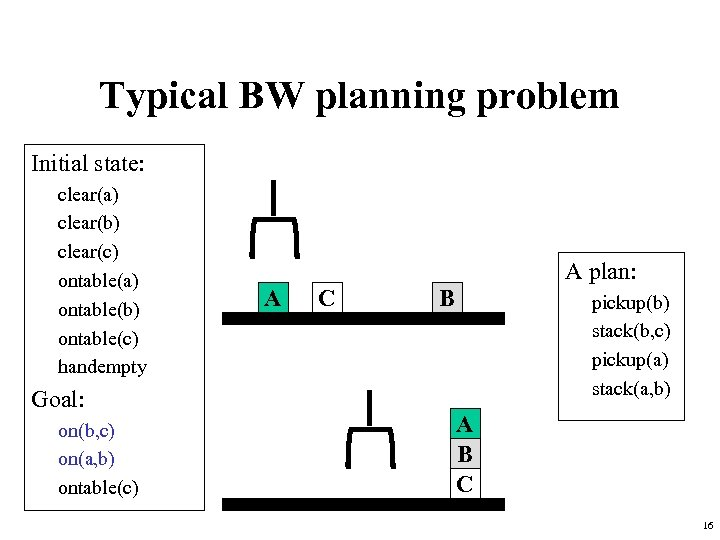Typical BW planning problem Initial state: clear(a) clear(b) clear(c) ontable(a) ontable(b) ontable(c) handempty Goal: on(b, c) on(a, b) ontable(c) A plan: A C B pickup(b) stack(b, c) pickup(a) stack(a, b) A B C 16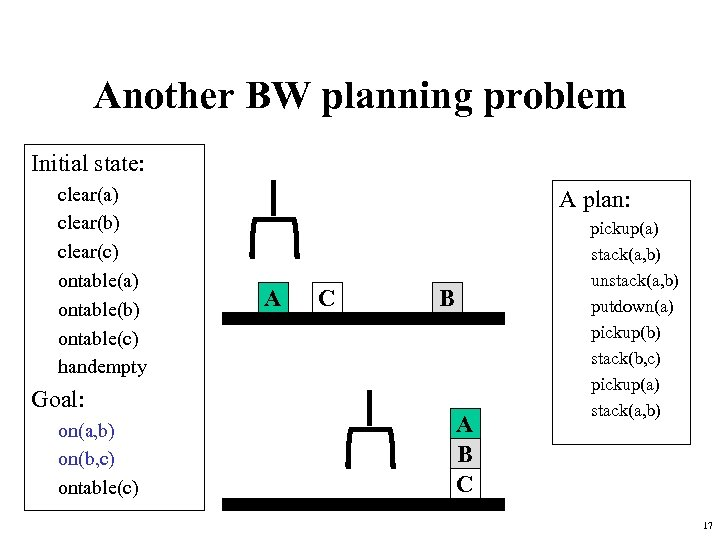Another BW planning problem Initial state: clear(a) clear(b) clear(c) ontable(a) ontable(b) ontable(c) handempty Goal: on(a, b) on(b, c) ontable(c) A plan: A C B A B C pickup(a) stack(a, b) unstack(a, b) putdown(a) pickup(b) stack(b, c) pickup(a) stack(a, b) 17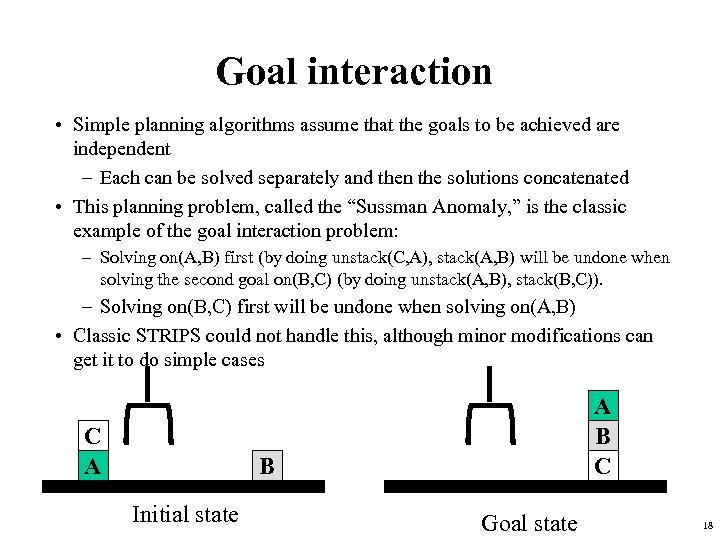Goal interaction • Simple planning algorithms assume that the goals to be achieved are independent – Each can be solved separately and then the solutions concatenated • This planning problem, called the “Sussman Anomaly, ” is the classic example of the goal interaction problem: – Solving on(A, B) first (by doing unstack(C, A), stack(A, B) will be undone when solving the second goal on(B, C) (by doing unstack(A, B), stack(B, C)). – Solving on(B, C) first will be undone when solving on(A, B) • Classic STRIPS could not handle this, although minor modifications can get it to do simple cases C A A B C B Initial state Goal state 18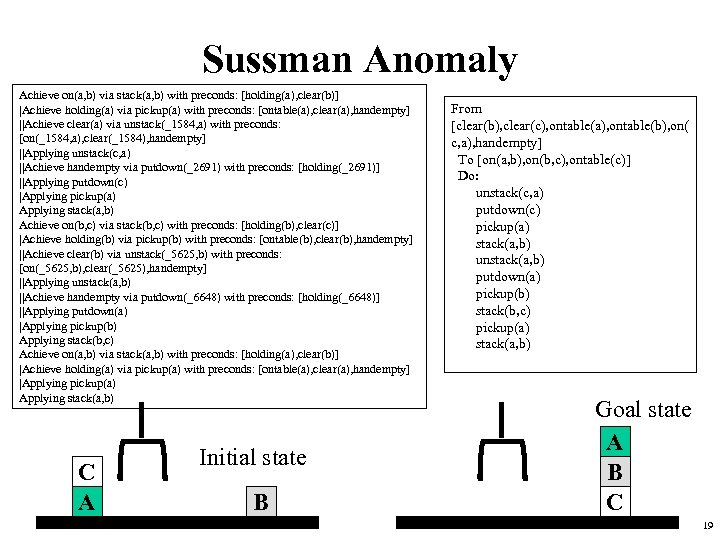Sussman Anomaly Achieve on(a, b) via stack(a, b) with preconds: [holding(a), clear(b)] |Achieve holding(a) via pickup(a) with preconds: [ontable(a), clear(a), handempty] ||Achieve clear(a) via unstack(_1584, a) with preconds: [on(_1584, a), clear(_1584), handempty] ||Applying unstack(c, a) ||Achieve handempty via putdown(_2691) with preconds: [holding(_2691)] ||Applying putdown(c) |Applying pickup(a) Applying stack(a, b) Achieve on(b, c) via stack(b, c) with preconds: [holding(b), clear(c)] |Achieve holding(b) via pickup(b) with preconds: [ontable(b), clear(b), handempty] ||Achieve clear(b) via unstack(_5625, b) with preconds: [on(_5625, b), clear(_5625), handempty] ||Applying unstack(a, b) ||Achieve handempty via putdown(_6648) with preconds: [holding(_6648)] ||Applying putdown(a) |Applying pickup(b) Applying stack(b, c) Achieve on(a, b) via stack(a, b) with preconds: [holding(a), clear(b)] |Achieve holding(a) via pickup(a) with preconds: [ontable(a), clear(a), handempty] |Applying pickup(a) Applying stack(a, b) C A Initial state B From [clear(b), clear(c), ontable(a), ontable(b), on( c, a), handempty] To [on(a, b), on(b, c), ontable(c)] Do: unstack(c, a) putdown(c) pickup(a) stack(a, b) unstack(a, b) putdown(a) pickup(b) stack(b, c) pickup(a) stack(a, b) Goal state A B C 19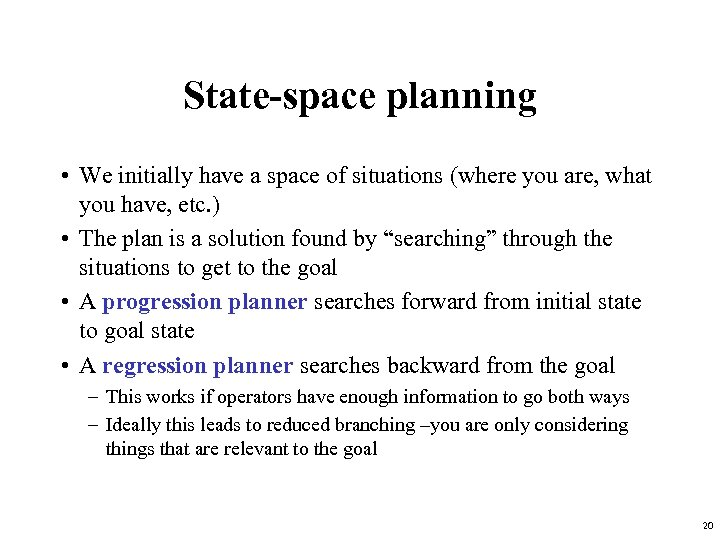State-space planning • We initially have a space of situations (where you are, what you have, etc. ) • The plan is a solution found by “searching” through the situations to get to the goal • A progression planner searches forward from initial state to goal state • A regression planner searches backward from the goal – This works if operators have enough information to go both ways – Ideally this leads to reduced branching –you are only considering things that are relevant to the goal 20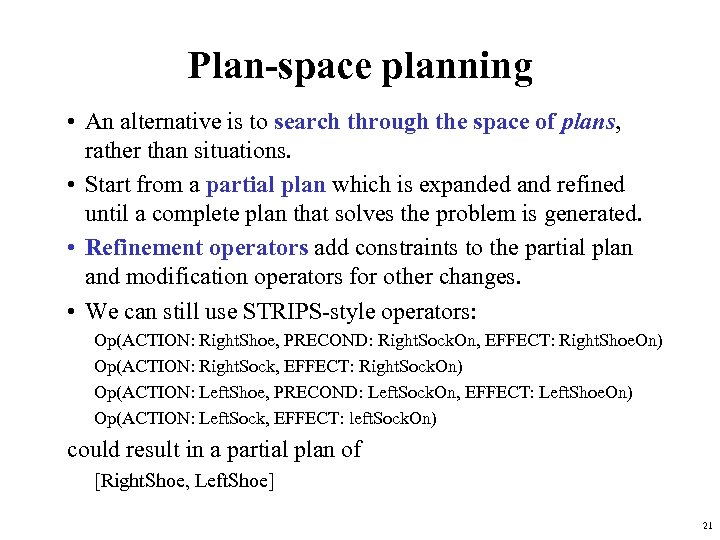Plan-space planning • An alternative is to search through the space of plans, rather than situations. • Start from a partial plan which is expanded and refined until a complete plan that solves the problem is generated. • Refinement operators add constraints to the partial plan and modification operators for other changes. • We can still use STRIPS-style operators: Op(ACTION: Right. Shoe, PRECOND: Right. Sock. On, EFFECT: Right. Shoe. On) Op(ACTION: Right. Sock, EFFECT: Right. Sock. On) Op(ACTION: Left. Shoe, PRECOND: Left. Sock. On, EFFECT: Left. Shoe. On) Op(ACTION: Left. Sock, EFFECT: left. Sock. On) could result in a partial plan of [Right. Shoe, Left. Shoe] 21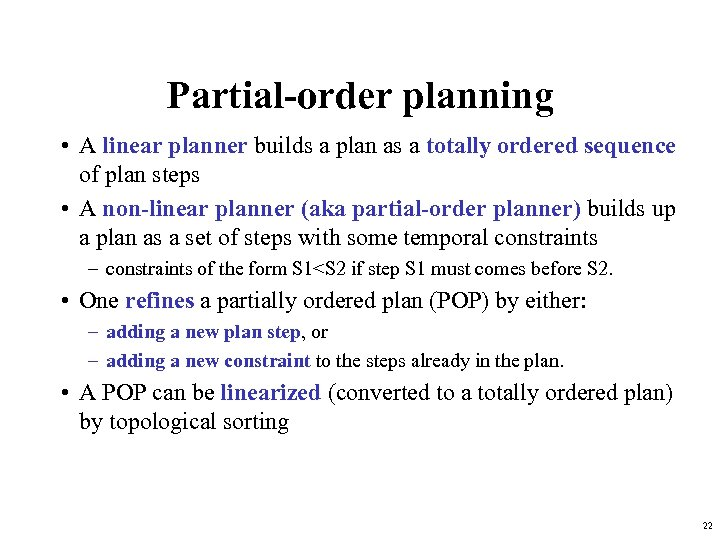Partial-order planning • A linear planner builds a plan as a totally ordered sequence of plan steps • A non-linear planner (aka partial-order planner) builds up a plan as a set of steps with some temporal constraints – constraints of the form S 1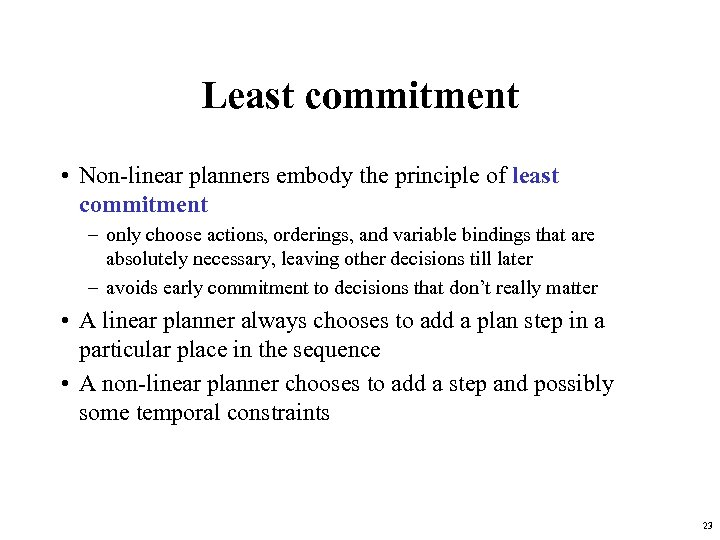Least commitment • Non-linear planners embody the principle of least commitment – only choose actions, orderings, and variable bindings that are absolutely necessary, leaving other decisions till later – avoids early commitment to decisions that don’t really matter • A linear planner always chooses to add a plan step in a particular place in the sequence • A non-linear planner chooses to add a step and possibly some temporal constraints 23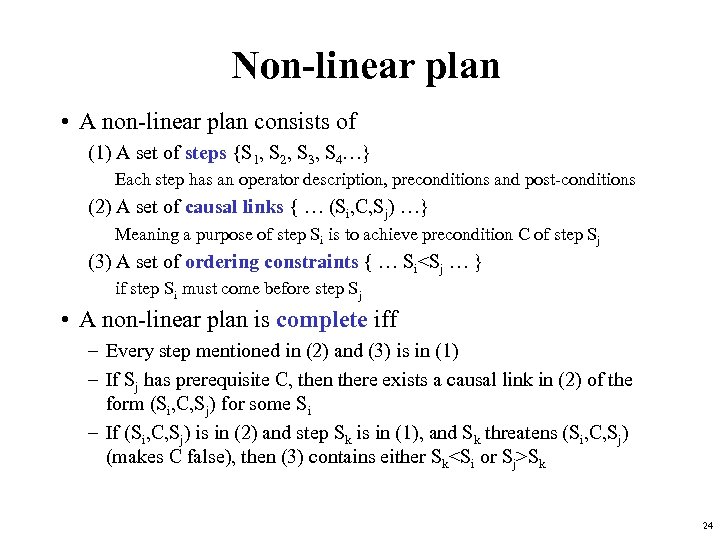Non-linear plan • A non-linear plan consists of (1) A set of steps {S 1, S 2, S 3, S 4…} Each step has an operator description, preconditions and post-conditions (2) A set of causal links { … (Si, C, Sj) …} Meaning a purpose of step Si is to achieve precondition C of step Sj (3) A set of ordering constraints { … SiSk 24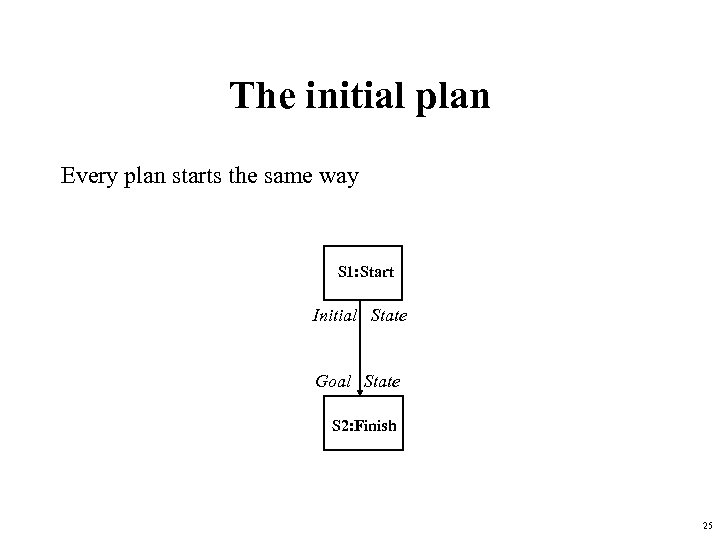The initial plan Every plan starts the same way S 1: Start Initial State Goal State S 2: Finish 25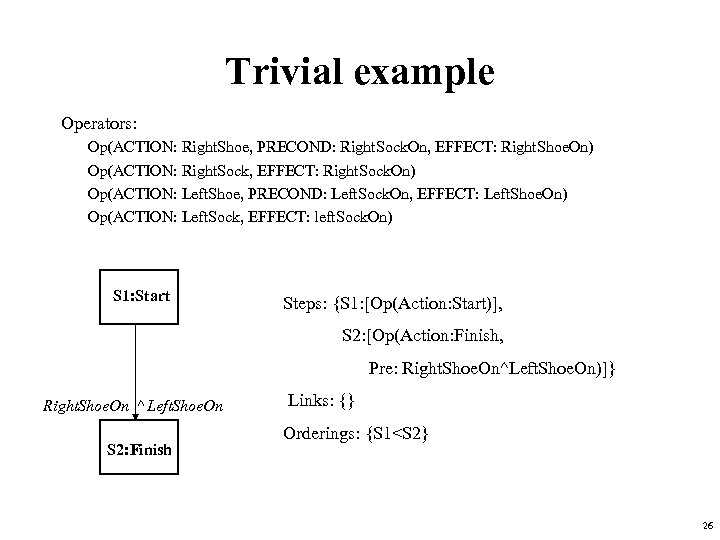Trivial example Operators: Op(ACTION: Right. Shoe, PRECOND: Right. Sock. On, EFFECT: Right. Shoe. On) Op(ACTION: Right. Sock, EFFECT: Right. Sock. On) Op(ACTION: Left. Shoe, PRECOND: Left. Sock. On, EFFECT: Left. Shoe. On) Op(ACTION: Left. Sock, EFFECT: left. Sock. On) S 1: Start Steps: {S 1: [Op(Action: Start)], S 2: [Op(Action: Finish, Pre: Right. Shoe. On^Left. Shoe. On)]} Right. Shoe. On ^ Left. Shoe. On S 2: Finish Links: {} Orderings: {S 1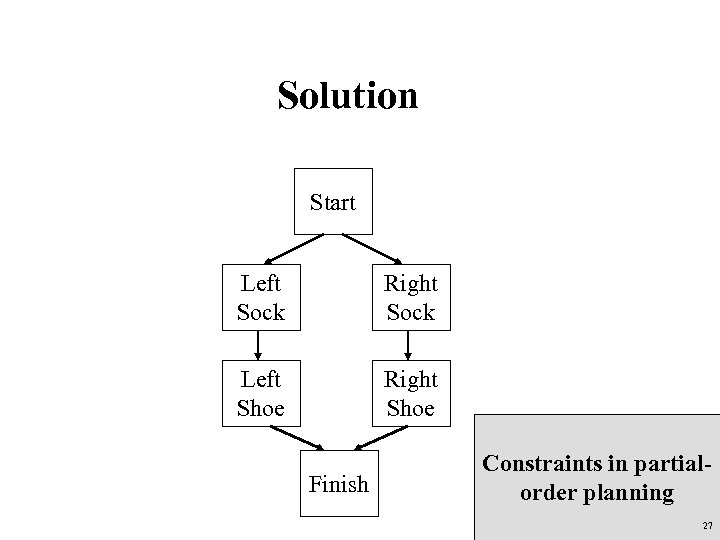Solution Start Left Sock Right Sock Left Shoe Right Shoe Finish Constraints in partialorder planning 27